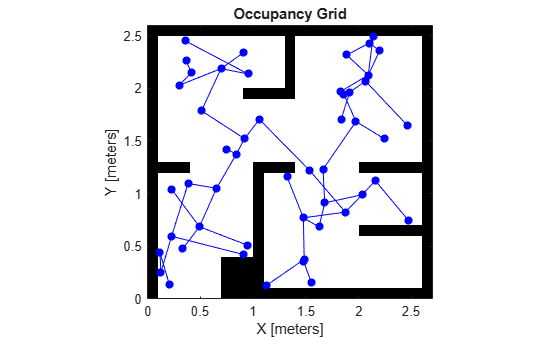# graphData

Retrieve graph as digraph object

Since R2022a

## Syntax

``graph = graphData(planner)``

## Description

example

````graph = graphData(planner)` retrieves graph as a `digraph` object. The `digraph` object is created when the `plannerPRM` object is created with default or user specified values for the `MaxNumNodes` and `MaxConnectionDistance` properties.```

## Examples

collapse all

Load an example map into the workspace, and use it to create an occupancy map with a resolution of 10 cells/meter.

```load("exampleMaps.mat","simpleMap"); map = occupancyMap(simpleMap,10);```

Create a state space object and update the state space bounds to match the map limits.

```ss = stateSpaceSE2; ss.StateBounds = [map.XWorldLimits; map.YWorldLimits; [-pi pi]];```

Create a state validator for the occupancy map using the state space object and set the validation distance.

```sv = validatorOccupancyMap(ss,Map=map); sv.ValidationDistance = 0.01;```

Create a probabilistic roadmap path planner object.

`planner = plannerPRM(ss,sv);`

Retrieve the network graph as a `digraph` object.

`graph = graphData(planner);`

Extract the nodes and edges from the graph.

```edges = table2array(graph.Edges); nodes = table2array(graph.Nodes);```

Plot the map and graph.

```show(sv.Map) hold on plot(nodes(:,1),nodes(:,2),"*",Color=[0 0 1],LineWidth=2) for i = 1:size(edges,1) % Samples states at distance 0.02 meters. states = interpolate(ss,nodes(edges(i,1),:), ... nodes(edges(i,2),:),0:0.02:1); plot(states(:,1),states(:,2),Color=[0 0 1]) end```## Input Arguments

collapse all

Path planner, specified as a `plannerPRM` object.

## Output Arguments

collapse all

Graph data, returned as a `digraph` object.

## Version History

Introduced in R2022a